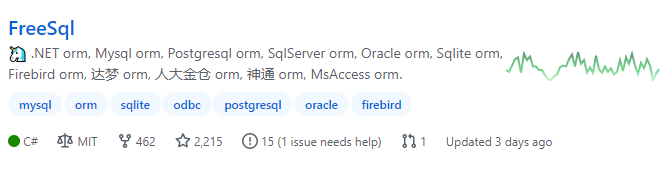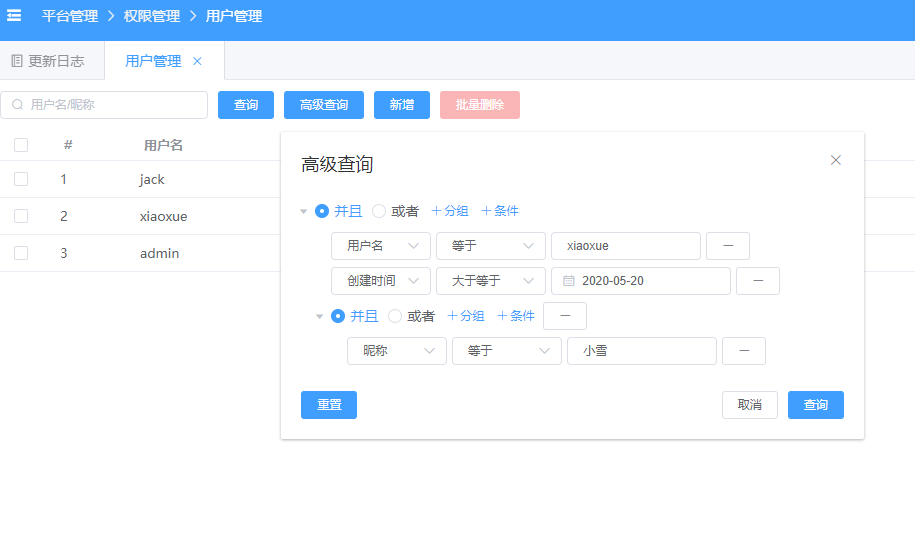# 写在开头

FreeSql 是 .NET 开源生态下的 ORM 轮子，转眼快两年了，说真的开源不容易（只有经历过才明白）。今天带点干货和湿货给大家，先说下湿货。

ctstone/csredis 项目于 2014 年停止了更新，到我手里完善的功能如下：

• 连接池
• 哨兵高可用
• 集群
• redis 2.8 以上的版本命令补充，包括 Geo、Stream
• 通讯协议 bug 修复

• 他去了 sqlsugar issues 发表，说要找出 FreeSql 抄袭 sqlsugar 的证据
• 他又去 fur issues 发表声援，说我黑他
• 他还去 csredis 原作者 issues 发布内容，企图告我的状

# 入戏准备

2018 年 12 月份开发 FreeSql 到现在，2200 颗星，500 Issues，200K 包下载量。说明还是有开发者关注和喜爱，只要有人关注，就不会停更不修 BUG 一说。大家有兴趣可以看看更新记录，看看我们的代码提交量，5400+ 单元测试不说非常多，个人觉得已经超过很多国产项目。

23个月了，FreeSql 还活着，而且生命力顽强见下图：FreeSql 是 .Net ORM，能支持 .NetFramework4.0+、.NetCore、Xamarin、XAUI、Blazor、以及还有说不出来的运行平台，因为代码绿色无依赖，支持新平台非常简单。目前单元测试数量：5400+，Nuget下载数量：200K+，源码几乎每天都有提交。值得高兴的是 FreeSql 加入了 ncc 开源社区：https://github.com/dotnetcore/FreeSql，加入组织之后社区责任感更大，需要更努力做好品质，为开源社区出一份力。

QQ群：4336577(已满)、8578575(在线)、52508226(在线)FreeSql 主要优势在于易用性上，基本是开箱即用，在不同数据库之间切换兼容性比较好。作者花了大量的时间精力在这个项目，肯请您花半小时了解下项目，谢谢。

FreeSql 整体的功能特性如下：

• 支持 CodeFirst 对比结构变化迁移；
• 支持 DbFirst 从数据库导入实体类；
• 支持 丰富的表达式函数，自定义解析；
• 支持 批量添加、批量更新、BulkCopy；
• 支持 导航属性，贪婪加载、延时加载、级联保存；
• 支持 读写分离、分表分库，租户设计；
• 支持 MySql/SqlServer/PostgreSQL/Oracle/Sqlite/Firebird/达梦/神通/人大金仓/翰高/MsAccess Ado.net 实现包，以及 Odbc 的专门实现包；

# 干货来了

1.5.0 -> 1.10.0 更新的重要功能如下：

});
Assert.Equal(\$@"SELECT concat('x',ifnull((a.Id + 1), ''),'z-',ifnull(date_format(a.CreateTime,'%Y%m'), ''),'',ifnull(a.Title, ''),'',ifnull(a.Title, ''),'') as1
FROM tb_topic a
WHERE (a.Id = {item.Id})", sql);


# 增加 lambda 表达式树解析子查询 ToList + string.Join() 产生 类似 group_concat 的效果；

v1.8.0+ string.Join + ToList 实现将子查询的多行结果，拼接为一个字符串，如："1,2,3,4"

fsql.Select<Topic>().ToList(a => new {
id = a.Id,
concat = string.Join(",", fsql.Select<StringJoin01>().ToList(b => b.Id))
});
//SELECT a.Id, (SELECT group_concat(b.Id separator ',')
//    FROM StringJoin01 b)
//FROM Topic a


# 增加 SqlExt 常用的自定义表达式树解析；

SqlExt.cs 定义了一些常用的表达式树解析，如下：

fsql.Select<T1, T2>()
.InnerJoin((a, b) => b.Id == a.Id)
.ToList((a, b) => new
{
Id = a.Id,
EdiId = b.Id,
over1 = SqlExt.Rank().Over().OrderBy(a.Id).OrderByDescending(b.EdiId).ToValue(),
case1 = SqlExt.Case()
.When(a.Id == 1, 10)
.When(a.Id == 2, 11)
.When(a.Id == 3, 12)
.When(a.Id == 4, 13)
.When(a.Id == 5, SqlExt.Case().When(b.Id == 1, 10000).Else(999).End())
.End(), //这里因为复杂才这样，一般使用三元表达式即可：a.Id == 1 ? 10 : 11
groupct1 = SqlExt.GroupConcat(a.Id).Distinct().OrderBy(b.EdiId).Separator("_").ToValue()
});


# 增加 ISelect/IInsert/IUpdate/IDelete CommandTimeout 方法设置命令超时；

fsql.Insert<items).CommandTimeout(60).ExecuteAffrows();

fsql.Delete<T>().Where(...).CommandTimeout(60).ExecuteAffrows();

fsql.Update<T>()
.Set(a => a.Clicks + 1)
.Where(...)
.CommandTimeout(60).ExecuteAffrows();

fsql.Select<T>().Where(...).CommandTimeout(60).ToList();


# 完善 WhereDynamicFilter 动态过滤查询DynamicFilterInfo dyfilter = JsonConvert.DeserializeObject<DynamicFilterInfo>(@"
{
""Logic"" : ""Or"",
""Filters"" :
[
{
""Field"" : ""Code"", ""Operator"" : ""NotContains"", ""Value"" : ""val1"",
""Filters"" : [{ ""Field"" : ""Name"", ""Operator"" : ""NotStartsWith"", ""Value"" : ""val2"" }]
},
{
""Field"" : ""Parent.Code"", ""Operator"" : ""Equals"", ""Value"" : ""val11"",
""Filters"" : [{ ""Field"" : ""Parent.Name"", ""Operator"" : ""Contains"", ""Value"" : ""val22"" }]
}
]
}");
fsql.Select<VM_District_Parent>().WhereDynamicFilter(dyfilter).ToList();
//SELECT a.""Code"", a.""Name"", a.""ParentCode"", a__Parent.""Code"" as4, a__Parent.""Name"" as5, a__Parent.""ParentCode"" as6
//FROM ""D_District"" a
//LEFT JOIN ""D_District"" a__Parent ON a__Parent.""Code"" = a.""ParentCode""
//WHERE (not((a.""Code"") LIKE '%val1%') AND not((a.""Name"") LIKE 'val2%') OR a__Parent.""Code"" = 'val11' AND (a__Parent.""Name"") LIKE '%val22%')


ISelect.WhereDynamicFilter 方法实现动态过滤条件（与前端交互），支持的操作符：

• Contains/StartsWith/EndsWith/NotContains/NotStartsWith/NotEndsWith：包含/不包含，like '%xx%'，或者 like 'xx%'，或者 like '%xx'
• Equal/NotEqual：等于/不等于
• GreaterThan/GreaterThanOrEqual：大于/大于等于
• LessThan/LessThanOrEqual：小于/小于等于
• Range：范围查询
• DateRange：日期范围，有特殊处理 value + 1
• Any/NotAny：是否符合 value 中任何一项（直白的说是 SQL IN）

# 增加 BeginEdit/EndEdit 批量编辑数据的功能；

[Fact]
public void BeginEdit()
{
fsql.Delete<BeginEdit01>().Where("1=1").ExecuteAffrows();
var repo = fsql.GetRepository<BeginEdit01>();
var cts = new[] {
new BeginEdit01 { Name = "分类1" },
new BeginEdit01 { Name = "分类1_1" },
new BeginEdit01 { Name = "分类1_2" },
new BeginEdit01 { Name = "分类1_3" },
new BeginEdit01 { Name = "分类2" },
new BeginEdit01 { Name = "分类2_1" },
new BeginEdit01 { Name = "分类2_2" }
}.ToList();
repo.Insert(cts);

repo.BeginEdit(cts); //开始对 cts 进行编辑

cts.Add(new BeginEdit01 { Name = "分类2_3" });
cts.Name = "123123";
cts.RemoveAt(1);

Assert.Equal(3, repo.EndEdit());
}
class BeginEdit01
{
public Guid Id { get; set; }
public string Name { get; set; }
}


INSERT INTO "BeginEdit01"("Id", "Name") VALUES('5f26bf07-6ac3-cbe8-00da-7dd74818c3a6', '分类2_3')

UPDATE "BeginEdit01" SET "Name" = '123123'
WHERE ("Id" = '5f26bf00-6ac3-cbe8-00da-7dd01be76e26')

DELETE FROM "BeginEdit01" WHERE ("Id" = '5f26bf00-6ac3-cbe8-00da-7dd11bcf54dc')


# 增加 父子表（树表）递归查询、删除功能；

• 方法1：表设计中只有 parent_id 字段，困扰：查询麻烦（本文可解决）；
• 方法2：表设计中冗余子级id便于查询，困扰：添加/更新/删除的时候需要重新计算；
• 方法3：表设计中存储左右值编码，困扰：同上；

public class Area
{
[Column(IsPrimary = true)]
public string Code { get; set; }

public string Name { get; set; }
public virtual string ParentCode { get; set; }

[Navigate(nameof(ParentCode))]
public Area Parent { get; set; }
[Navigate(nameof(ParentCode))]
public List<Area> Childs { get; set; }
}


fsql.Select<Area>().Where(a => a.Parent.Parent.Parent.Name == "中国").First();


fsql.Select<Area>().Where(a => a.Childs.AsSelect().Any(c => c.Name == "北京")).First();


fsql.Delete<Area>().Where("1=1").ExecuteAffrows();
var repo = fsql.GetRepository<Area>();
repo.DbContextOptions.NoneParameter = true;
repo.Insert(new Area
{
Code = "100000",
Name = "中国",
Childs = new List<Area>(new[] {
new Area
{
Code = "110000",
Name = "北京",
Childs = new List<Area>(new[] {
new Area{ Code="110100", Name = "北京市" },
new Area{ Code="110101", Name = "东城区" },
})
}
})
});


var t1 = fsql.Select<Area>().ToTreeList();
Assert.Single(t1);
Assert.Equal("100000", t1.Code);
Assert.Single(t1.Childs);
Assert.Equal("110000", t1.Childs.Code);
Assert.Equal(2, t1.Childs.Childs.Count);
Assert.Equal("110100", t1.Childs.Childs.Code);
Assert.Equal("110101", t1.Childs.Childs.Code);


fsql.Select<Area>()
.Where(a => a.Name == "中国")
.AsTreeCte()
.ToDelete()
.ExecuteAffrows(); //删除 中国 下的所有记录


fsql.Select<Area>()
.Where(a => a.Name == "中国")
.AsTreeCte()
.ToUpdate()
.Set(a => a.IsDeleted, true)
.ExecuteAffrows(); //软删除 中国 下的所有记录


(可选) pathSelector 路径内容选择，可以设置查询返回：中国 -> 北京 -> 东城区
(可选) up false(默认)：由父级向子级的递归查询，true：由子级向父级的递归查询
(可选) pathSeparator 设置 pathSelector 的连接符，默认：->
(可选) level 设置递归层级

var t2 = fsql.Select<Area>()
.Where(a => a.Name == "中国")
.AsTreeCte() //查询 中国 下的所有记录
.OrderBy(a => a.Code)
.ToTreeList(); //非必须，也可以使用 ToList（见姿势二）
Assert.Single(t2);
Assert.Equal("100000", t2.Code);
Assert.Single(t2.Childs);
Assert.Equal("110000", t2.Childs.Code);
Assert.Equal(2, t2.Childs.Childs.Count);
Assert.Equal("110100", t2.Childs.Childs.Code);
Assert.Equal("110101", t2.Childs.Childs.Code);
// WITH "as_tree_cte"
// as
// (
// SELECT 0 as cte_level, a."Code", a."Name", a."ParentCode"
// FROM "Area" a
// WHERE (a."Name" = '中国')

// union all

// SELECT wct1.cte_level + 1 as cte_level, wct2."Code", wct2."Name", wct2."ParentCode"
// FROM "as_tree_cte" wct1
// INNER JOIN "Area" wct2 ON wct2."ParentCode" = wct1."Code"
// )
// SELECT a."Code", a."Name", a."ParentCode"
// FROM "as_tree_cte" a
// ORDER BY a."Code"


var t3 = fsql.Select<Area>()
.Where(a => a.Name == "中国")
.AsTreeCte()
.OrderBy(a => a.Code)
.ToList();
Assert.Equal(4, t3.Count);
Assert.Equal("100000", t3.Code);
Assert.Equal("110000", t3.Code);
Assert.Equal("110100", t3.Code);
Assert.Equal("110101", t3.Code);
//执行的 SQL 与姿势一相同


var t4 = fsql.Select<Area>()
.Where(a => a.Name == "中国")
.AsTreeCte(a => a.Name + "[" + a.Code + "]")
.OrderBy(a => a.Code)
.ToList(a => new {
item = a,
level = Convert.ToInt32("a.cte_level"),
path = "a.cte_path"
});
Assert.Equal(4, t4.Count);
Assert.Equal("100000", t4.item.Code);
Assert.Equal("110000", t4.item.Code);
Assert.Equal("110100", t4.item.Code);
Assert.Equal("110101", t4.item.Code);
Assert.Equal("中国", t4.path);
Assert.Equal("中国 -> 北京", t4.path);
Assert.Equal("中国 -> 北京 -> 北京市", t4.path);
Assert.Equal("中国 -> 北京 -> 东城区", t4.path);
// WITH "as_tree_cte"
// as
// (
// SELECT 0 as cte_level, a."Name" || '[' || a."Code" || ']' as cte_path, a."Code", a."Name", a."ParentCode"
// FROM "Area" a
// WHERE (a."Name" = '中国')

// union all

// SELECT wct1.cte_level + 1 as cte_level, wct1.cte_path || ' -> ' || wct2."Name" || '[' || wct2."Code" || ']' as cte_path, wct2."Code", wct2."Name", wct2."ParentCode"
// FROM "as_tree_cte" wct1
// INNER JOIN "Area" wct2 ON wct2."ParentCode" = wct1."Code"
// )
// SELECT a."Code" as1, a."Name" as2, a."ParentCode" as5, a.cte_level as6, a.cte_path as7
// FROM "as_tree_cte" a
// ORDER BY a."Code"


# 写在最后

FreeSql 使用最宽松的开源协议 MIT https://github.com/dotnetcore/FreeSql，完全可以商用，文档齐全。QQ群：4336577(已满)、8578575(在线)、52508226(在线)

2.0 版本意见正在登记中：https://github.com/dotnetcore/FreeSql/issues/469

posted @ 2020-10-22 03:32  nicye  阅读(4820)  评论(109编辑  收藏